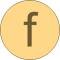# isEqual

Determine if two Real vectors are numerically identical# Information

This information is part of the Modelica Standard Library maintained by the Modelica Association.

#### Syntax

```Vectors.isEqual(v1, v2);
Vectors.isEqual(v1, v2, eps=0);
```

#### Description

The function call "`Vectors.isEqual(v1, v2)`" returns true, if the two Real vectors v1 and v2 have the same dimensions and the same elements. Otherwise the function returns false. Two elements e1 and e2 of the two vectors are checked on equality by the test "abs(e1-e2) ≤ eps", where "eps" can be provided as third argument of the function. Default is "eps = 0".

#### Example

```  Real v1 = {1, 2, 3};
Real v2 = {1, 2, 3, 4};
Real v3 = {1, 2, 3.0001};
Boolean result;
algorithm
result := Vectors.isEqual(v1,v2);     // = false
result := Vectors.isEqual(v1,v3);     // = false
result := Vectors.isEqual(v1,v1);     // = true
result := Vectors.isEqual(v1,v3,0.1); // = true
```